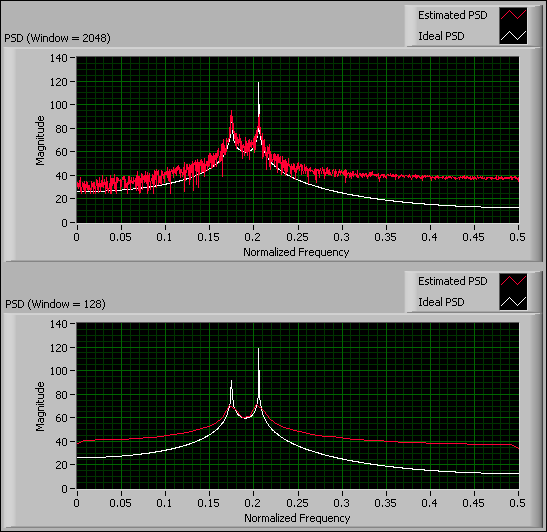# Using the Welch Method (Advanced Signal Processing Toolkit)

LabVIEW 2014 Advanced Signal Processing Toolkit Help

Edition Date: June 2014

Part Number: 372656C-01

»View Product InfoDownload Help (Windows Only)

The Welch method reduces the variance of the periodogram method by averaging. This method first divides a time series into overlapping subsequences by applying a window to each subsequence and then averaging the periodogram of each subsequence.

The length of the applied window controls the trade-off between bias and variance of the resulting power spectral density (PSD). The following figure shows the resulting PSD for a signal from a system with two pairs of poles at about 0.17 and 0.21 Hz, when the window length is 2048 and 128 for the top and bottom plots, respectively.In the PSD (Window = 2048) graph, a large window generates a PSD with small bias in the locations of the two peaks, as you can see that the magnitudes of the peaks in the Estimated PSD plot almost equal the magnitudes of the peaks in the Ideal PSD plot. However, a large window results in a coarse PSD plot, as shown by the general fuzziness in the Estimated PSD plot.

In the PSD (Window = 128) graph, you can see that a small window generates a smooth Estimated PSD plot. However, a small window can lead to large bias, as you can see that the magnitudes of the peaks in the Estimated PSD plot are different from the magnitudes of the peaks in the Ideal PSD plot.

Use the TSA Welch VI to compute the PSD of a time series.